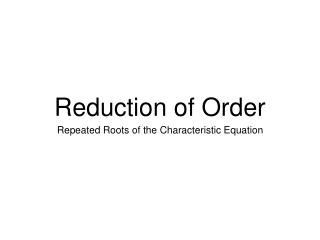DownloadDownload PresentationReduction of Order

# Reduction of Order

Download Presentation## Reduction of Order

- - - - - - - - - - - - - - - - - - - - - - - - - - - E N D - - - - - - - - - - - - - - - - - - - - - - - - - - -
##### Presentation Transcript

1. Reduction of Order • Repeated Roots of the Characteristic Equation

2. So far… • We’ve Learned How To Solve Second Order Linear Homogeneous ODEs with Constant Coefficients • Characteristic Equation • We’ve seen distinct real roots, complex conjugate roots

3. Characteristic Equation Gives the Characteristic Equation Case 1: If roots are real and distinct, and General solution: Case 2: If roots are complex, and General solution:

4. Characteristic Equation Gives the Characteristic Equation What if there’s only one root, and ?

5. Characteristic Equation Gives the Characteristic Equation What if there’s only one root, Then we know one solution: How do we find another?

6. Reduction of Order If we have a linear homogeneous second order equation and we know one solution How do we find another?

7. Reduction of Order One solution In general, finding solutions is difficult

8. Reduction of Order One solution Guess second solution has the form: Insert guess into ODE

9. Reduction of Order One solution Guess second solution has the form: Insert guess into ODE

10. Reduction of Order One solution Guess second solution has the form: Insert guess into ODE

11. Reduction of Order One solution Guess second solution has the form: Insert guess into ODE

12. Reduction of Order One solution Guess second solution has the form: Insert guess into ODE

13. Reduction of Order One solution Guess second solution has the form: Insert guess into ODE

14. Reduction of Order One solution Guess second solution has the form: Insert guess into ODE

15. Reduction of Order One solution Guess second solution has the form: Insert guess into ODE Can be solved with a first order linear ODE

16. Reduction of Order One solution Guess second solution has the form: Insert guess into ODE Make a clever “renaming”

17. Reduction of Order One solution Guess second solution has the form: Insert guess into ODE Make a clever “renaming”

18. Reduction of Order One solution Guess second solution has the form: Insert guess into ODE First Order Linear ODE

19. Reduction of Order One solution Guess second solution has the form: Insert guess into ODE First Order Linear ODE Second solution is Use Wronskian to establish a Fundamental Set of Solutions

20. Important Example Characteristic Equation: One Solution

21. Important Example One Solution

22. Important Example One Solution

23. Important Example One Solution

24. Important Example One Solution

25. Important Example One Solution Second Solution

26. Important Example One Solution: Second Solution: Wronskian: Wronskian: Form a Fundamental Set of Solutions General Solution:

27. This Holds in General Gives the Characteristic Equation Only one root, and

28. This Holds in General One solution: Determine Reduction of Order Equation

29. This Holds in General One solution: Determine Reduction of Order Equation

30. This Holds in General One solution: Determine Reduction of Order Equation

31. This Holds in General One solution: Determine Reduction of Order Equation

32. This Holds in General One solution: Determine Reduction of Order Equation Second Solution:

33. This Holds in General If Characteristic Equation Has only one root, General Solution takes the form:

34. Summary • We now know how to solve Second Order Linear Homogeneous with Constant Coefficients: • Characteristic Equation • Distinct Real Roots, Complex Conjugate Roots, One (Repeated) Root • If we have a Second Order Linear Homogeneous Equation and one solution, use Reduction of Order to find second solution.

35. Questions?